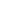#MTH-1002 Arithmetic Operations is a Course

## MTH-1002 Arithmetic Operations

Self-paced

### Full course description

Course Description

Arithmetic has four basic operations: addition, subtraction, multiplication, and division. Each operation has its own symbols, terminology, and rules.

By the end of this course, you will be able to

• Name the four basic arithmetic operations

• Identify the symbols for the four basic operations

• Identify the arithmetic comparator symbols

• Define the terminology used with the four basic operations

• Perform math problems using the correct order of operations

Estimated completion time (hours): 1.4